The graph which will be representing all the equilibrium concentrations for the reaction N2O4(g) $\underset{}{\overset{}{⇌}}$ 2NO2 (g) will be : (the concentrations of N2O4 (g) and of NO (g) for which the following reaction will be at equilibrium will lie

(1)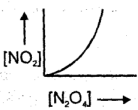(2)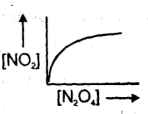(3)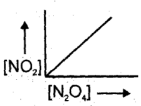(4)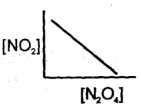High Yielding Test Series + Question Bank - NEET 2020

Difficulty Level:

In a basic aqueous solution chloromethane undergoes a substitution reaction in which Cl is replaced byOH as. CH3CI (aq) + OH$\underset{}{\overset{}{⇌}}$ CH3OH(aq) + Cl (aq) The equilibrium constant of above reaction C = 1 × 10–6. If a solution is prepared by mixing equal volumes of 0.1 M CH3CI and 0.2 M NaOH (100% dissociated) then [OH] concentration at equilibrium in mixture will be:

(1) 0.1 M

(2) 0.5 M

(3) 0.2 M

(4) 0.05 M

High Yielding Test Series + Question Bank - NEET 2020

Difficulty Level:

For the chemical equilibrium, CaCO3(s) $\stackrel{}{⇌}$ CaO(s) + CO2 (g) ΔHf° can be determined from which one of the following plots ?

(1)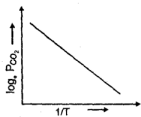(2)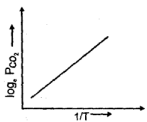(3)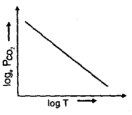(4)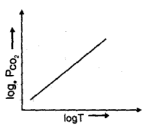High Yielding Test Series + Question Bank - NEET 2020

Difficulty Level:

At a certain temperature the following equilibrium is established, CO(g) + N02(g) $\stackrel{}{⇌}$ CO2(g)+ NO(g)One mole of each of the four gas is mixed in one litre container and the reaction is allowed to reach equilibrium state. When excess of baryta water (Ba(OH)2) is added to the equilibrium mixture, the weight of white ppt (BaCO3) obtained is 236.4 gm. The equilibrium constant Kc of the reaction is (Ba = 137)

(1) 1.2

(2) 2.25

(3) 2.1

(4) 3.6

High Yielding Test Series + Question Bank - NEET 2020

Difficulty Level:

At temperature T, the compound AB2 (g) dissociates according to the reaction, 2AB2 (g) $\stackrel{}{⇌}$ 2 AB(g) + B2(g). With a degree of dissociation x, which is small compared with unity. Deduce the expression for x in terms of the equilibrium constant, Kp and the total pressure, P.

(1)

(2)

(3)

(4)

High Yielding Test Series + Question Bank - NEET 2020

Difficulty Level:

Equilibrium constant for the given reaction is kc = 1020 at temperature 300 K,A(s) + 2B (aq.) $\stackrel{}{⇌}$ 2C (s) + D (aq.)K = 1020 The equilibrium conc. of B starting with mixture of 1 mole of A and 1/3 mole/litre of B at 300 K is

(1) $~4×{10}^{–11}$

(2) $~2×{10}^{–10}$

(3) $~2×{10}^{–11}$

(4) $~{10}^{–10}$

High Yielding Test Series + Question Bank - NEET 2020

Difficulty Level:

10l box contain O3 and O2 at equilibrium at 2000 K. The ΔG° = –534.52 kJ at 8 atm equilibrium pressure.The following equilibrium is present in the container. 2O3(g) $\stackrel{}{⇌}$ 3O2(g). The partial pressure of O3 will be (In 10 = 2.3, R = 8.3 Jmole–1K–1):

(1) 8 ×104

(2) 22.62 × 10-7

(3) 9.71 × 10-6

(4) 9.71 × 10-2

High Yielding Test Series + Question Bank - NEET 2020

Difficulty Level:

Solid ammonium carbamate dissociates to give ammonia and carbon dioxide as follows:

NH2COONH4(s) $\stackrel{}{⇌}$ 2 NH3(g) + CO2(g) At equilibrium, ammonia is added such that partial pressures of NH3 now equals the original total pressure. Calculate the ratio of the total pressures now to the original total pressure.

(1) $\frac{31}{27}$

(2) $\frac{60}{40}$

(3) $\frac{31}{9}$

(4) $\frac{62}{27}$

Concept Questions :-

KP/KC - Factors
High Yielding Test Series + Question Bank - NEET 2020

Difficulty Level:

The reactions, PCl5 (g) $\stackrel{}{⇌}$ PCl3(g) + Cl2(g) and Cl2(g) $\stackrel{}{⇌}$ CO2(g) + Cl2(g) are simultaneously in equilibrium in an equilibrium box at constant volume. A few moles of CO(g) are later introduced into the vessel. After some time, the new equilibrium concentration of

(1) PCl5 will remain unchanged

(2) Cl2 will be greater

(3) PCl5 will become less

(4) PCl5 will become greater

Concept Questions :-

Le Chatelier
High Yielding Test Series + Question Bank - NEET 2020

Difficulty Level:

In the Haber process for the industrial manufacture of ammonia involving the reaction, N2 + 3H2 $\stackrel{}{⇌}$ 2NH3 at 200 atm pressure in the presence of a catalyst, temperature of about 500°C. This is considered as optimum temperature for the process because

(1) yield is maximum at this temperature

(2) catalyst is active only at this temperature

(3) energy needed for the reaction is easily obtained at this temperature

(4) rate of the catalytic reaction is fast enough while the yield is also appreciable for this exothermic reaction at this temperature.

Concept Questions :-

Le Chatelier